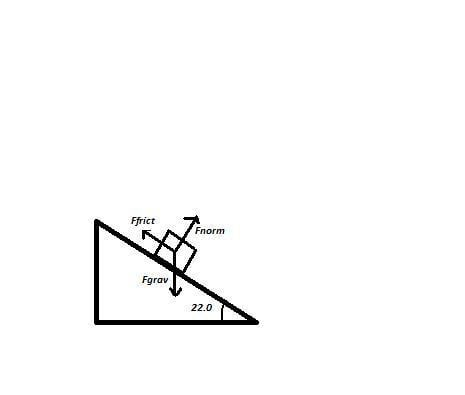# Determining the Acceleration (Inclined Plane Problem)

• yandereni

## Homework Statement

A crate lies on a plane tilted at an angle θ = 22.0° to the horizontal, with μkin = 0.12.
a.) determine the acceleration of the crate as it slides down the plane
b.) if the carton starts from rest 9.30m up the plane from its base, what will be the carton's speed when it reaches the bottom?

this is the most confusing so far...

## Homework Equations

ΣFhorizontal = Ffrict+Fgrav(sin22) = ma
ΣFvertical = Fnorm + Fgrav(cos22) = ma
Vfinal2= VInitial2 + 2a(displacement)## The Attempt at a Solution

ΣFhorizontal = (0.12)(Fnorm)+Fgrav(sin22) = ma
= 0.12Fn + 0.375Fg=ma
= 0.12(0.279Fg + ma) = ma
= 0.111Fg + 0.12ma = ma
= 0.111Fg = 0.88ma
=Fg = 7.928ma
ΣFvertical = Fnorm + Fgrav(cos22) = ma
= Fn - 0.927Fg = ma
=Fn=0.927Fg+ma
= Fn= 0.927(7.928ma)
=Fn = 7.349ma

since I didnt really know what to do next..
7.928ma/7.349ma= 1.079
so i assumed that was the acceleration

then...

Vfinal2 = (0m/s)2 + (2)(1.079m/s2)(9.3m)
Vfinal2=(20.07m/s)
(Vfinal2)1/2=(20.07m/s)1/2
Vfinal=4.480m/s

•DAVID H HAINDONGO
Your approach to part b) is correct, but the value for the acceleration is wrong.

To be honest, I couldn't really understand what you were doing in part a).

The first step is to find the normal force acting on the block. If we call this ##F_n##, can you work out what ##F_n## is?

You have written subscript "horizontal" where you should call it "down the slope". Similarly, you wrote "vertical" where you should have "normal to the slope".

Don't forget that friction acts opposite to the direction of motion. So with the block sliding down the slope here, friction will be acting up the slope.

ΣFvertical = Fnorm + Fgrav(cos22) = ma
As NascentOxygen posted, you mean Fnormal, not Fvertical, but what is a in that equation? Yes, I know it's an acceleration, but what acceleration exactly?